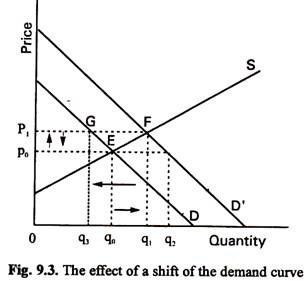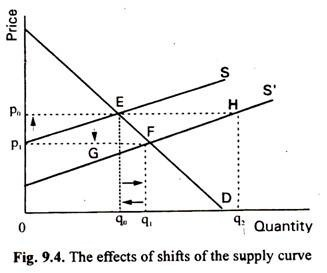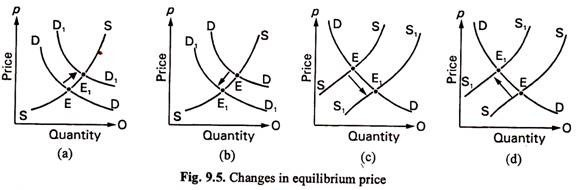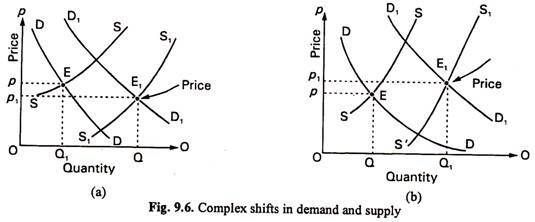# Shifts in Demand and Supply (With Diagram)

Let us make an in-depth study of the shifts in demand and supply.

So long we have examined how markets work when the only factor that influences demand and supply is the price of the commodity under consideration.

To do this, we made use of the ceteris paribus assump­tion and held all other factors which influence demand and supply constant. We may now relax the assumption in order to see how changes in the conditions of supply and demand (i.e., changes in other variables) affect market price and quantity.

It may be repeated that changes in the conditions of demand or supply cause shifts of the demand or supply curve to a new posi­tion. Each curve can shift either to the right or to the left. A rightward shift refers to an increase in demand or supply. The impli­cation is that a larger quantity is demanded, or supplied, at each market price. A leftward shifts refers to a decrease in demand or supply. It means that less is demanded or supplied, at each price. We may now refer to the following four laws of supply and demand.

#### Four “Laws” of Supply and Demand:

Since both the supply and demand curves can shift in either of the two directions, we have to consider four cases of changes in demand and supply. These cases are so important and universal in nature that they are often called ‘laws of supply and demand’.

These laws are derived for free markets that we are considering. Such markets have the following features:

(i) the demand curve is downward sloping,

(ii) the supply curve is upward sloping,

(iii) the buyers and sellers are price-takers and

(iv) the buyers and sellers are maximizers.

The laws of demand and supply are applicable only when these conditions hold. If anyone these conditions are not applicable the laws may not hold.

1. A Rise in Demand:

Let us first consider a rise in demand as in Fig. 9.3. The original demand curve is D and the supply is S. Here p0 is the original equili­brium price and q0 is the equilibrium quantity.We may now consider a change in the conditions of demand such as a rise in the income of buyers. If the income of the buyers rises the market demand curve for carrots will shift to right to D’. This implies that consumers will now be willing to buy a larger quantity at every price.

Thus at the original price P0 they will now be eager to buy q2 units. So excess demand develops in the market. This excess demand q2-q0 creates market forces which cause the equilibrium price to rise. The process will continue until a new equilibrium is reached as at point F where the new demand curve intersects the old supply curve. The net result is a rise in market price to p1. The quantity sold also increase from q0 to q1 in this new equilibrium situation.

So we first consider (1) rightward shift of the demand curve (i.e., a rise in the demand for a commodity) causes an increase in the equilibrium price and quantity (as is shown by the arrows in Fig. 9.3).

2. A Fall in Demand:

Next we may consider the effect of a fall in demand. Demand may fall due to changes in the conditions of demand. If, for example, there is a fall in the price of a substitute for the commodity under consideration, consumers may want to buy smaller quantities at every price.

Suppose D’ in Fig. 9.3 is the original de­mand curve. Now the original price and quan­tity are p1 and q1, respectively. Suppose a fall in demand leads to a leftward shift of the .demand curve. The new demand curve is D. So an excess supply q1– q3 (=FG) develops in the market.

As a result of the operation of the market forces price falls. The new equilibrium price is p0. The new equilibrium quantity is q0. So we reach the second conclu­sion a leftward shift of the demand curve (i.e., a fall in the demand for a commodity) causes a decrease in the equilibrium price and quantity.

3. An Increase in Supply:

In Fig. 9.4 we consider the effect of a shift in the supply curve. Here S and D are original supply and demand curves. The two curves meet at point E. So p0 and q0 are the original equilibrium price and quantity. We may now examine the effect of a change in the condi­tions of supply.Such a change increases the quantities that producers are prepared to offer for sale at each price. For example, there was a rightward shift of the supply curve due to increase in the productivity of factors of production, caused by technological advance.

The Green Revolution which has occurred in India is an example of such a change. Techno­logical progress has the effect of reducing the cost of production. As a result, a larger quan­tity (qt instead of q0) is offered for sale at a lower price (p1 instead of p0). This happened in the computer industry in the late 90’s.

An increase in supply implies that a larger quantity is offered for sale at the same price (q2, instead of q0 at p0) or the same quantity at a lower price (as point G indicates). In other words, an excess of supply of q0 q2 (=EH) develops at the original price p0. It sets in motion market forces which cause the price to fall.

Since there is not much demand for their product, producers find it difficult to sell the entire output at the original price. They start charging lower price. Consumers know about it and start paying a lower price. Conse­quently price starts falling and it ultimately reaches the value p1. At this new price the equilibrium quantity is q1. Thus we reach the third conclusion a rightward shift of the supply curve (i.e., an increase in the supply of a commodity) causes a fall in the equilibrium price and an increase in equilibrium quantity.

4. A Decrease in Supply:

Finally, we may examine the effect of a rise in the price of a factor, such as wages in a unionized industry. As a result, total cost will rise and the sellers will be willing to offer a smaller quantity for sale at each price. In this case, the original supply curve is S’. Equi­librium price and quantity are p1 and q1. Now the supply curve shifts to left. The new supply curve is S.

At the original equilibrium price p1, the quantity offered for sale is zero but the quantity demanded is still q1. So the entire quantity demanded (viz., q1) is excess demand. This excess demand sets in motion market forces which tend to raise price. The process continues until and unless the new equilibrium price p0 is reached.

At this price the quantity supplied and demanded are equated at q0. Thus we reach the fourth and final conclusion a leftward shift in the supply curve (i.e., a decrease in the supply of a commodity) leads to an increase in the equilibrium price and a fall in equilibrium quantity.

#### Changes in Market Prices: Recap:

From our discussion so far we discover four possibilities for change in market price as Fig. 9.5 shows. In this figure we consider all the four possibilities of changes in demand and supply.

Ceteris paribus, an increase in demand will bring about an extension of supply so that more is supplied at a higher price [Fig. 9.5(a)]. A fall in demand leads to a contraction of supply with a smaller quantity purchased at a lower price [Fig. 9.5(b)]. Conversely, an increase in supply causes an extension of demand so that more is bought at a lower price [Fig. 9.5(c)] and a decrease in supply causes a contraction of demand so that less is purchased at a higher price [Fig. 9.5(d)].#### Complex Changes:

So long we were able to reach may firm conclusions regarding shifts of supply and demand curves because we stuck to the ceteris paribus assumption, i.e., we considered only one change at a time. But, in practice, it is possible for two factors to vary at the same time. Suppose, there is a large rise in the demand for mangoes because of a rise in per capita income of the people.

This may be followed by an unexpected bumper crop of mangoes. What will be the final effect of such changes on the equilibrium price? The answer can be found from both the following dia­grams. However, although both the quantity demanded and quantity supplied increase in each case, in Fig. 9.6(a) the market price falls and in Fig. 9.6(b) it rises.Thus, when multiple shifts in demand and supply curves are considered price may rise or fall depending on the two magnitudes of changes a change in demand and a change in supply.

Suppose, one is asked to consider the effect of a number of changes in the demand and supply of a particular product. It is clear from Fig. 9.6(a) and 9.6(b) that no firm conclusion can be reached unless both changes move in the same direction; for example, an increase in supply and a decrease in demand at the same time will definitely lower the equili­brium price.

The solution lies in explaining one change at a time. For instance, fast explain the effect of an increase in demand and draw a diagram to illustrate it. Then explain the effect of the increase in supply by drawing another diagram. So one must always stick to the rule of explaining one change at a time unless one is having precise details of demand and supply.

It is also possible to show that if the supply curve shifts to the left due to bad crop and the demand curve shifts to the right due to rising per capita income, the same quantity will be offered for sale at a higher price. In this case price will be higher as a result of both types of changes but the equilibrium quantity will be the same.

Sometimes shifts of curves and movements cause confusion as the following state­ment shows:

‘An increase in income causes demand to rise. The rise in demand causes an increase in price. The increase in price causes an increase in supply, which pushes price back towards its original level.’

What is the mistake in this quotation? There is no doubt that an increase in income certainly shifts the demand curve to the right. As a result of a rise in demand, price rises. It is also true that the rise in price tends to increase the quantity supplied. But the rest of the statement is wrong. How can supply increase along the same supply curve (because there is no shift of the supply curve)? In fact, there is an increase in quantity supplied along the same supply curve.

The mistake lies in confusing a movement along the supply curve, as a result of a change in price, which does occur, with a shift in the supply curve which does not occur.